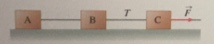# Problem: Three identical blocks connected by ideal strings are being pulled along a horizontal frictionless surface by a horizontal force F. The magnitude of the tension in the string between blocks B and C is T = 3.00 N. Assume that each block has mass m = 0.400 kg. A. What is the tension in the string between block A and block B? B. What is the magnitude of the force?

###### FREE Expert Solution

We can get the coefficient of friction from the tension between sliding B and C due to frictional force A and B.

T = FA + FB

T = μmAg + μmBg = 2μmg

μ = T/2mg = 3.00/(2)(0.400)(9.81) = 0.38

97% (242 ratings)###### Problem Details

Three identical blocks connected by ideal strings are being pulled along a horizontal frictionless surface by a horizontal force F. The magnitude of the tension in the string between blocks B and C is T = 3.00 N. Assume that each block has mass m = 0.400 kg.A. What is the tension in the string between block A and block B?

B. What is the magnitude of the force?